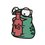# Prove this inequality

Problem 1.

Prove that the inequality

$\prod_{k=1}^n \left( 1+\frac{1}{3k-2}\right)>\displaystyle \sqrt{3n+1}$

is true $\forall n \in \mathbb{Z}^{+}$

=============================================

Proof:

Lemma 1: if the real numbers $M, m, c>0$ satisfy $M > m$, then

\begin{aligned}\frac{m}{M} &< \frac{m+c}{M+c} \\ \frac{M}{m} &> \frac{M+c}{m+c} \end{aligned}

The lemma is self-explanatory if we think of $\frac{m}{M}$ as the "saltiness" of a glass of salty water with total mass $M$, and $m$ is the mass of the salt. By increasing the numerator and denominator by the same amout (adding salt of mass $c$ in the glass) the "saltiness" increases.

By Lemma 1,

$\prod_{k=1}^n \left( 1+\frac{1}{3k-2}\right) = \prod_{k=1}^n \left(\frac{3k-1}{3k-2}\right) > \prod_{k=1}^n \left(\frac{3k}{3k-1}\right)>\prod_{k=1}^n \left(\frac{3k+1}{3k}\right)$

Therefore,

\begin{aligned}\left(\prod_{k=1}^n \left(\frac{3k-1}{3k-2}\right)\right)^2> \prod_{k=1}^n \left(\frac{3k}{3k-1}\right)\prod_{k=1}^n \left(\frac{3k+1}{3k}\right)&=\prod_{k=1}^n \left(\frac{3k+1}{3k-1}\right)\\&=(3n+1)\prod_{k=1}^{n-1}(3k+1) \prod_{k=1}^n \left( 3k-1\right)^{-1}\\&=(3n+1)\prod_{k=1}^{n}(3(k-1)+1) \prod_{k=1}^n \left( 3k-1\right)^{-1} \\& = (3n+1)\prod_{k=1}^n \left(\frac{3k-2}{3k-1}\right) \end{aligned}

Hence

$\left(\prod_{k=1}^n \left(\frac{3k-1}{3k-2}\right)\right)^3 > 3n+1$

since $\sqrt{x}$ is an increasing function

$\prod_{k=1}^n \left( 1+\frac{1}{3k-2}\right) > \sqrt{3n+1}$

and this completes our proof.

=============================================Note by Daniel Xiang
3 years, 3 months ago

This discussion board is a place to discuss our Daily Challenges and the math and science related to those challenges. Explanations are more than just a solution — they should explain the steps and thinking strategies that you used to obtain the solution. Comments should further the discussion of math and science.

When posting on Brilliant:

• Use the emojis to react to an explanation, whether you're congratulating a job well done , or just really confused .
• Ask specific questions about the challenge or the steps in somebody's explanation. Well-posed questions can add a lot to the discussion, but posting "I don't understand!" doesn't help anyone.
• Try to contribute something new to the discussion, whether it is an extension, generalization or other idea related to the challenge.

MarkdownAppears as
*italics* or _italics_ italics
**bold** or __bold__ bold
- bulleted- list
• bulleted
• list
1. numbered2. list
1. numbered
2. list
Note: you must add a full line of space before and after lists for them to show up correctly
paragraph 1paragraph 2

paragraph 1

paragraph 2

[example link](https://brilliant.org)example link
> This is a quote
This is a quote
    # I indented these lines
# 4 spaces, and now they show
# up as a code block.

print "hello world"
# I indented these lines
# 4 spaces, and now they show
# up as a code block.

print "hello world"
MathAppears as
Remember to wrap math in $$ ... $$ or $ ... $ to ensure proper formatting.
2 \times 3 $2 \times 3$
2^{34} $2^{34}$
a_{i-1} $a_{i-1}$
\frac{2}{3} $\frac{2}{3}$
\sqrt{2} $\sqrt{2}$
\sum_{i=1}^3 $\sum_{i=1}^3$
\sin \theta $\sin \theta$
\boxed{123} $\boxed{123}$